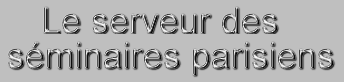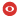Statut Confirmé Série IPN-X Domaines hep-ph Date Jeudi 25 Avril 2019 Heure 11:00 Institut CPHT Salle Salle de conférence Louis Michel, bât. 6 Nom de l'orateur Semenov-Tian-Shansky Prenom de l'orateur Kirill Addresse email de l'orateur Institution de l'orateur St. Petersburg, INP & Ioffe Phys. Tech. Inst. Titre Leading logarithms in massless effective field theories in $D = 2$ and elliptic functions Résumé We discuss the non-linear recurrence relations for the coefficients of Leading Logarithms (LLs) in massless Effective Field Theories (EFTs). These relations can be worked out relying on the fundamental properties of quantum field theory amplitudes: analyticity, unitarity and crossing symmetry and generalize the renormalization group technique for the case of non-renormalizable EFTs. We put particular emphasize on the $D=2$ case and address the mathematical properties of the relevant class of non-linear recurrence relations. We present a number of highly non-trivial solutions in terms of elliptic functions. We introduce the class of quasi-renormalizable quantum field theories for which the summation of leading logarithmic corrections for $2 \rightarrow 2$ scattering amplitudes results in manifestation of an infinite number of the Landau poles. Finally, we present explicit examples of quasi-renormalizable QFTs in $D=2$. Numéro de preprint arXiv Commentaires Fichiers attachés 2019-04-25_slides.pdf (2192069 bytes)Pour obtenir l' affiche de ce séminaire : [ Postscript | PDF ]

 [ Annonces ]    [ Abonnements ]    [ Archive ]    [ Aide ]    [ JavaScript requis ] [ English version ]